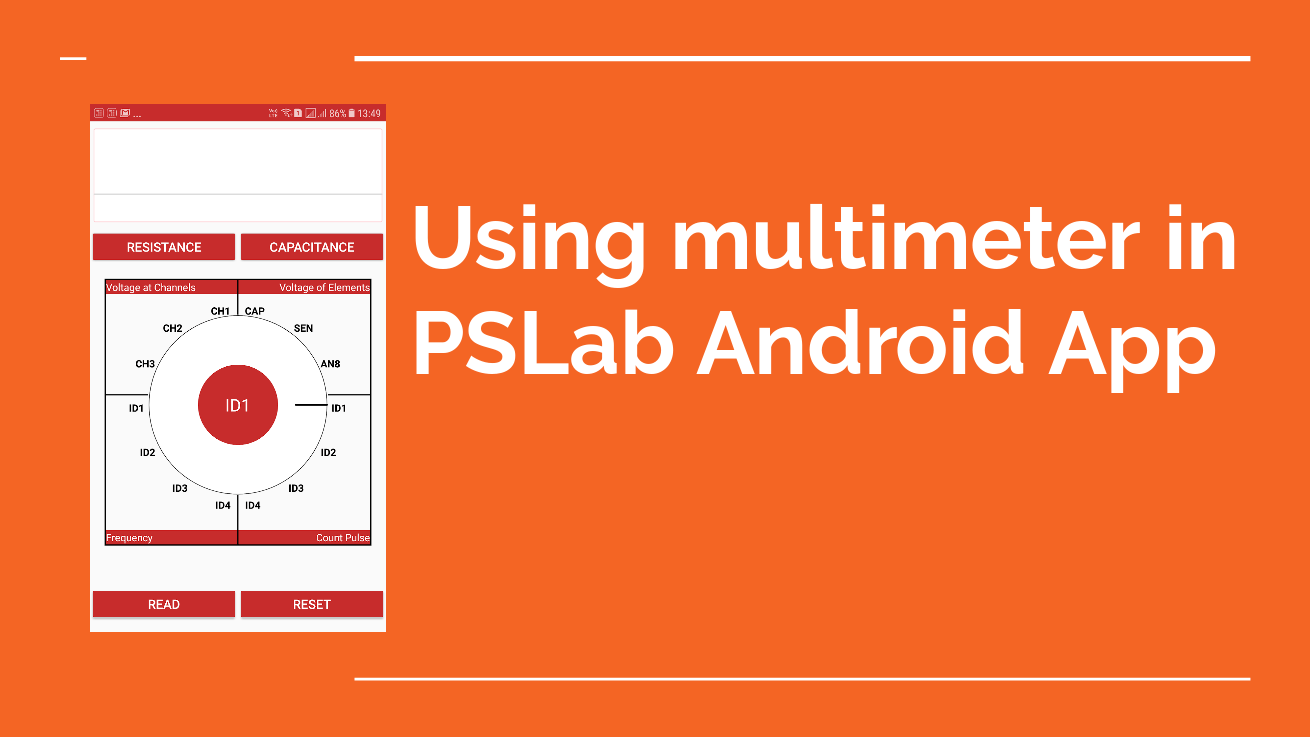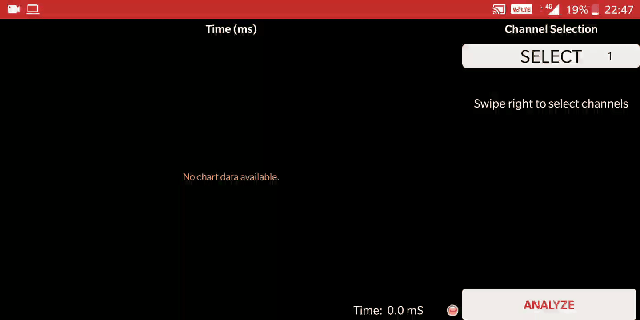# Using Multimeter in PSLab Android Application

The Pocket Science Lab as we all know is on the verge of development and new features and UI are added almost every day. One such feature is the implementation of multimeter which I have also discussed in my previous blogs.Figure (1) : Screenshot of the multimeter

But although many functionality of multimeter such as resistance measurement are working perfectly, there are still various bugs in the multimeter. This blog is dedicated to using multimeter in the android app.

### Using the multimeterFigure (2): Screenshot showing guide of multimeter

Figure (2) shows the guide of the multimeter, i.e how basic basic elements such as resistance and voltage are measured using the multimeter. The demonstration of measuring the resistance and voltage are given below.

#### Measuring the resistance

The resistance is measure by connecting the SEN pin to the positive end of resistor and the GND pin to the negative end of resistor and then clicking the RESISTANCE button.Figure (3) : Demonstration of resistance measurement

#### Measuring the voltage

To measure the voltage as said in the Guide, directly connect the power source to the the channel pins, although currently only the CH3 pin will show the most accurate results, work is going on other channel improvisation as well.Figure (4) : Demonstration of Voltage measurement

And thus this is how the multimeter is used is used in the android app.  Of course there are still many many features such as capacitance measurements which are yet to be implemented and the work is going on them

Resources: